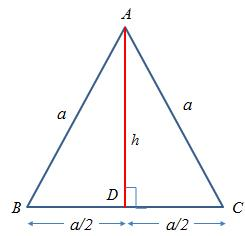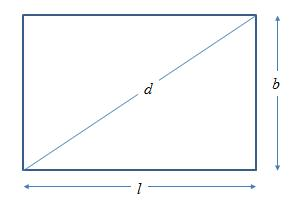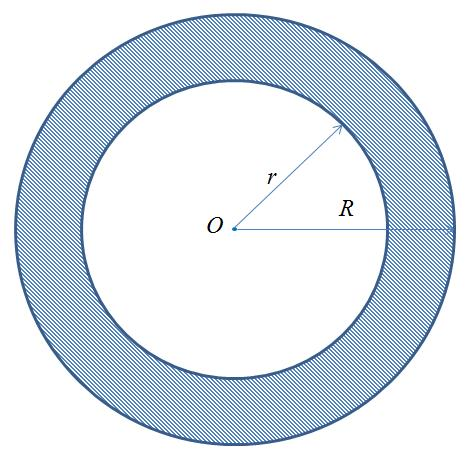TRIANGLES

Perimeter of a TriangleIf$a, \ b, \ c\$  are the lengths of the sides of any triangle. Then:

1. Perimeter of a triangle$= (a+b+c)$ units
2. Area of the triangle$= \sqrt{(s(s-a)(s-b)(s-c)} \ units.$

where$\displaystyle s=\frac{1}{2}(a+b+c)$.This is also known as$Heron's \ Formula$

Area of a Triangle

Refer to the adjoining figure. If b is the base and h is the height, then$\displaystyle Area\ of\ triangle = \frac{1}{2}(base \times height) = \frac{1}{2}(b \times h)$

Note: If you could take any side as the base, then the corresponding height is the

would be the length of the perpendicular to this side from the opposite vertex.

Area of Right Angled Triangle

Let the$\triangle ABC$, with$\angle B = 90^o$. Please refer to the adjoining figure.$\displaystyle Area \ of \ triangle = \frac{1}{2}(BC \times AB)$

Area of an Equilateral triangleIn an equilateral triangle, all three sides are equal.

Let us say the side$= a$  unit.

1. Height of an equilateral triangle =$\displaystyle \frac{\sqrt{3}}{2}a$  units
2. Area of an equilateral triangle with side$\displaystyle a \ is\ \frac{\sqrt{3}}{2}a^2$ units

RECTANGLE & SQUARE

Perimeter and Area of RectangleIf the sides of a rectangle are$l$ units and$b$units (refer to adjoining figure), then

1. Perimeter of rectangle$= 2(l+b)$ units
2. Area of rectangle$= (l \times b)$  sq. units
3. Diagonal of a rectangle$(d) = \sqrt{(l^2+b^2)}$ units

Perimeter and Area of a SquareIf the sides of a square is$a$ units, then

1. Perimeter$= 4a$ units
2. Area$= a^2$ units
3. Diagonal of a square$= a \sqrt{2}$ units

PARALLELOGRAM, RHOMBUS AND TRAPEZIUM

Area of a ParallelogramLet ABCD be a Parallelogram with base b  and height h units. Let AC be the diagonal. Refer to the adjoining figure.$Area = (b \times h)$ sq. units.

Area of a RhombusPlease refer to the adjoin diagram. Let$d1 \ and\ d2$ are the diagonals of the Rhombus. We know that the diagonals intersect at right angles and bisect each other.$\displaystyle Area\ of\ Rhombus= \frac{1}{2} \times (product\ of\ diagonals) = \frac{1}{2} \times (AC \times DB)$ sq. units

Area of a TrapeziumPlease refer to the adjoining figure.$AB \parallel DC$$\displaystyle Area\ of\ Trapezium \ ABCD \\= \frac{1}{2} \times (sum \ of \ the \ parallel \ sides) \times (distance\ between\ them) \\= \frac{1}{2} \times (AB + DC) \times (h) \ sq. \ units$

CIRCLE

Circumference and Area of a CircleLet the circle be of radius r

Circumference of the circle$= 2\pi r = \pi d$

Area of the circle$= \pi r^2$

Area of a Ring (shaded area)

Refer to the adjoining figure. The radius of the larger circle is R and that of the smaller circle is r.  Area of the ring is the shaded area.$Area\ of\ the\ ring=\pi R^2 - \pi r^2 = \pi(R^2-r^2) = \pi(R+r)(R-r)$